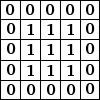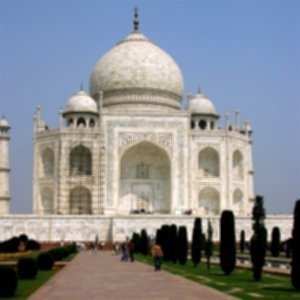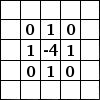# Understanding Convolutional Neural Networks for NLP

Dive into the world of Convolution Neural Networks (CNN), learn how they work, how to apply them for NLP, and how to tune CNN hyperparameters for best performance.

When we hear about Convolutional Neural Network (CNNs), we typically think of Computer Vision. CNNs were responsible for major breakthroughs in Image Classification and are the core of most Computer Vision systems today, from Facebook’s automated photo tagging to self-driving cars.

More recently we’ve also started to apply CNNs to problems in Natural Language Processing and gotten some interesting results. In this post I’ll try to summarize what CNNs are, and how they’re used in NLP. The intuitions behind CNNs are somewhat easier to understand for the Computer Vision use case, so I’ll start there, and then slowly move towards NLP.

### What is Convolution?

The for me easiest way to understand a convolution is by thinking of it as a sliding window function applied to a matrix. That’s a mouthful, but it becomes quite clear looking at a visualization:Fig.1 Convolution with 3×3 Filter. Source: http://deeplearning.stanford.edu/wiki/index.php/Feature_extraction_using_convolution

Imagine that the matrix on the left represents an black and white image. Each entry corresponds to one pixel, 0 for black and 1 for white (typically it’s between 0 and 255 for grayscale images). The sliding window is called a kernel, filter, or feature detector. Here we use a 3×3 filter, multiply its values element-wise with the original matrix, then sum them up. To get the full convolution we do this for each element by sliding the filter over the whole matrix.

You may be wondering wonder what you can actually do with this. Here are some intuitive examples.

#### Averaging each pixel with its neighboring values blurs an image:#### Taking the difference between a pixel and its neighbors detects edges:

(To understand this one intuitively, think about what happens in parts of the image that are smooth, where a pixel color equals that of its neighbors: The additions cancel and the resulting value is 0, or black. If there’s a sharp edge in intensity, a transition from white to black for example, you get a large difference and a resulting white value)The GIMP manual has a few other examples. To understand more about how convolutions work I also recommend checking out Chris Olah’s post on the topic.

### What are Convolutional Neural Networks?

Now you know what convolutions are. But what about CNNs? CNNs are basically just several layers of convolutions with nonlinear activation functions like ReLU or tanh applied to the results. In a traditional feedforward neural network we connect each input neuron to each output neuron in the next layer. That’s also called a fully connected layer, or affine layer. In CNNs we don’t do that. Instead, we use convolutions over the input layer to compute the output. This results in local connections, where each region of the input is connected to a neuron in the output.Each layer applies different filters, typically hundreds or thousands like the ones showed above, and combines their results. There’s also something something called pooling (subsampling) layers, but I’ll get into that later. During the training phase, a CNN automatically learns the values of its filtersbased on the task you want to perform. For example, in Image Classification a CNN may learn to detect edges from raw pixels in the first layer, then use the edges to detect simple shapes in the second layer, and then use these shapes to deter higher-level features, such as facial shapes in higher layers. The last layer is then a classifier that uses these high-level features.There are two aspects of this computation worth paying attention to: Location Invariance and Compositionality. Let’s say you want to classify whether or not there’s an elephant in an image. Because you are sliding your filters over the whole image you don’t really care where the elephant occurs. In practice,  pooling also gives you invariance to translation, rotation and scaling, but more on that later. The second key aspect is (local) compositionality. Each filter composes a local patch of lower-level features into higher-level representation. That’s why CNNs are so powerful in Computer Vision. It makes intuitive sense that you build edges from pixels, shapes from edges, and more complex objects from shapes.

By subscribing you accept KDnuggets Privacy Policy

By subscribing you accept KDnuggets Privacy Policy